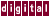## 4.5.7 /each_invariant_if_growth, /eiifg, (/eiifg=20)

When a loop contains an IF statement whose condition does not change from one iteration to another (loop-invariant), the same test must be repeated for every iteration. The code can often be made more efficient by floating the IF outside the loop and putting the THEN and ELSE sections into their own loops.

This gets more complicated when there is other code in the loop, since a copy of it must be included in both the THEN and ELSE loops, for example:

```code_example>
for ( i = ...) {
section-1
if ( ) {
section-2
}
else
{
section-3
}
section-4
enddo
```

Becomes:

```      if  ( ) {
for ( i = ...) {
section-1
section-2
section-4
}
}
else
{
for ( i = ...) {
section-1
section-3
section-4
}
}
```

When sections 1 and 4 are large, the extra code generated can slow a program down through cache contention, extra paging, and so on, more than the reduced number of IF tests speed it up. The ```/each_ invariant_if_growth``` qualifier provides a maximum size (in number of lines of executable code) of sections 1 and 4 which KAP will try to float an invariant IF outside a loop.

This can be controlled on a loop-by-loop basis with the `C*\$* each_invariant_if_growth` (<integer>) directive. The total amount of additional code generated in a program unit through invariant-IF floating can be limited with the `/max_invariant_if_growth` qualifier.

Previous Page Next Page Contents Index
Command-Line Qualifiers